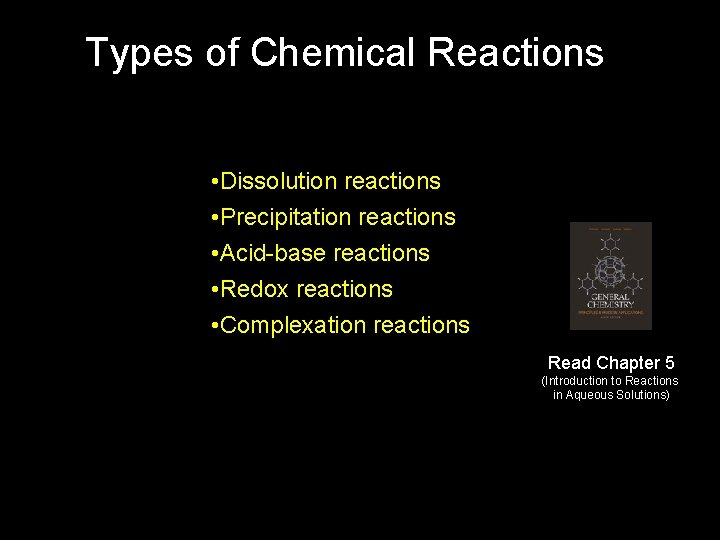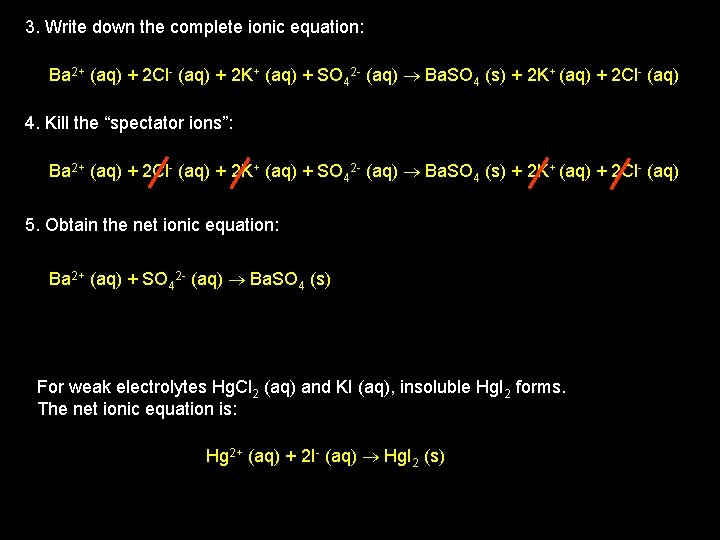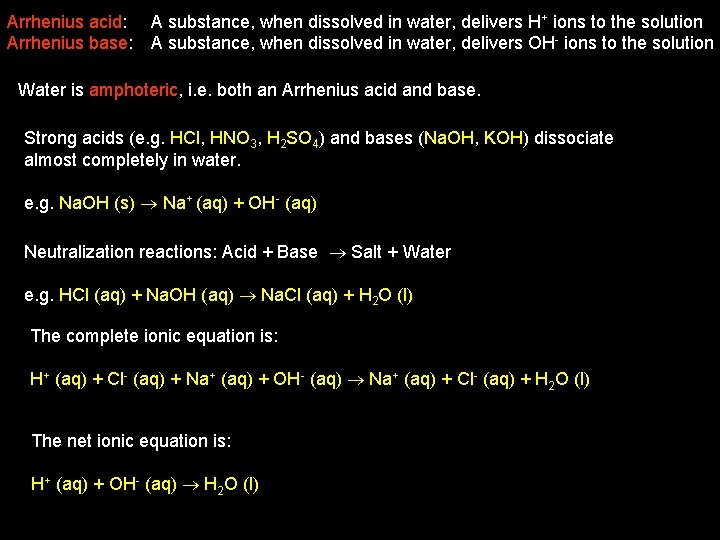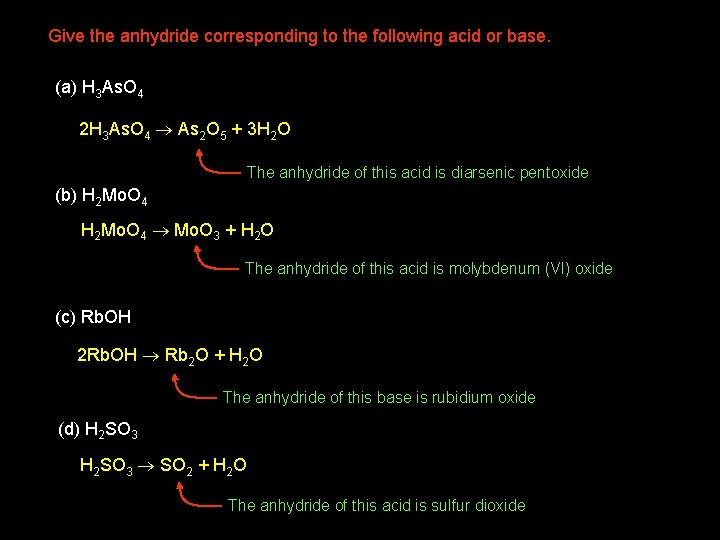# Types of Chemical Reactions Dissolution reactions Precipitation reactions

• Slides: 11Types of Chemical Reactions • Dissolution reactions • Precipitation reactions • Acid-base reactions • Redox reactions • Complexation reactions Read Chapter 5 (Introduction to Reactions in Aqueous Solutions)Dissolution Reactions Solute Dissolves in solvent to produce a solution Usually driven by solvent-solute attractive interactions e. g. Dissociation (ionization): Na. Cl (s) Na+ (aq) + Cl- (aq) The lattice energy is Na. Cl (s) Na+ (g) + Cl- (g), Compare to dissolution energy 797 k. J mol-1 Why does Mg. SO 4 lower the temperature of water? Mg. SO 4 Mg 2+ + SO 42 - Depends on lattice energy (requires energy, endothermic) (H 2 O)n n. H 2 O Solvent-solvent interactions (overcoming H-bonds, slightly endothermic) Mg 2+ + H 2 O Mg 2+ (aq) Formation of aquo complex and solvation sphere (exothermic) SO 42 - + H 2 O SO 42 - (aq) Solvation (exothermic) E (solvation) < E (lattice) dissolution is overall endothermic More energy is required to break up Mg. SO 4 than is released by solvation. Why does this happen?Dissolution Reactions Ionic compounds dissolve in water to give electrolytes • Resulting electrolyte has higher conductivity than pure water • Incomplete production of ions during dissolution yields “weak” electrolytes • Oppositely charged aquated ions can form ion pairs (reduces conductivity) Dissolution of non-electrolytes substances – driven by dipolar interactions e. g. Fructose C 6 H 12 O 6 (s) C 6 H 12 O 6 (aq) Solubility – usually two substances are only partially miscible Ether (1. 36 g of water per 100 g of ether) 25 o. C Water (6. 41 g of ether per 100 g of water) Solubility limit – largest amount that can dissolve in a given amount of solvent at a particular temperaturePrecipitation Reactions When solubility limit is exceeded solids usually precipitate. Can occur if (i) Solvent evaporates (ii) Changing solvent composition e. g. Na. Cl in water, 36 g/100 g Na. Cl in ethanol, 0. 12/100 g adding ethanol to Na. Cl (aq) can precipitate Na. Cl (s) (iii) Changing temperature For slightly soluble substance Ba. Cl 2 mixed with very soluble K 2 SO 4 Ba. Cl 2 (aq) + K 2 SO 4 (aq) Ba. SO 4 (s) + 2 KCl (aq) Determining the “net ionic equation” 1. Write down all the ions involved: Ba 2+ (aq) + 2 Cl- (aq) + 2 K+ (aq) + SO 42 - (aq) 2. Identify the “insoluble product/s: Ba. SO 4 (s)3. Write down the complete ionic equation: Ba 2+ (aq) + 2 Cl- (aq) + 2 K+ (aq) + SO 42 - (aq) Ba. SO 4 (s) + 2 K+ (aq) + 2 Cl- (aq) 4. Kill the “spectator ions”: Ba 2+ (aq) + 2 Cl- (aq) + 2 K+ (aq) + SO 42 - (aq) Ba. SO 4 (s) + 2 K+ (aq) + 2 Cl- (aq) 5. Obtain the net ionic equation: Ba 2+ (aq) + SO 42 - (aq) Ba. SO 4 (s) For weak electrolytes Hg. Cl 2 (aq) and KI (aq), insoluble Hg. I 2 forms. The net ionic equation is: Hg 2+ (aq) + 2 I- (aq) Hg. I 2 (s)Predicting precipitations; some general rules: (a) Check the solubilities of the compounds formed by switching partners (b) If either is insoluble (or slightly soluble) precipitation occurs (c) If both insoluble two precipitation reactions (d) If both solution no precipitation An aqueous solution of Na. NO 3 is mixed with a solution of KCl. The reaction, Na. NO 3 + KCl Na. Cl + KNO 3, is anticipated, but nothing happens. Explain. All compounds: Na. Cl, KNO 3, Na. NO 3, KCl Na+ (aq) + NO 3 - (aq) + K+ (aq) + Cl- (aq) Na+ (aq) + Cl- (aq) + K+ (aq) + NO 3 - (aq) NR (no reaction) Acid-Base Reactions Autoionization is an intrinsic property of water: H 2 O (l) H+ (aq) + OH- (aq) water always contains very small amounts of H+ and OH- ions (a weak electrolyte)Arrhenius acid: A substance, when dissolved in water, delivers H+ ions to the solution Arrhenius base: A substance, when dissolved in water, delivers OH- ions to the solution Water is amphoteric, i. e. both an Arrhenius acid and base. Strong acids (e. g. HCl, HNO 3, H 2 SO 4) and bases (Na. OH, KOH) dissociate almost completely in water. e. g. Na. OH (s) Na+ (aq) + OH- (aq) Neutralization reactions: Acid + Base Salt + Water e. g. HCl (aq) + Na. OH (aq) Na. Cl (aq) + H 2 O (l) The complete ionic equation is: H+ (aq) + Cl- (aq) + Na+ (aq) + OH- (aq) Na+ (aq) + Cl- (aq) + H 2 O (l) The net ionic equation is: H+ (aq) + OH- (aq) H 2 O (l)Neutralization reactions: Acid + Base Salt + Water There are exceptions: NH 3 (aq) + HCl (aq) NH 4 Cl (aq), no water Bases The only soluble hydroxides are Ba(OH)2 and alkali metal hydroxides (e. g. Na. OH, KOH) The others donate OH- poorly, i. e. weak bases. However, weak bases will neutralize acids (will dissolve in acidic solution) e. g. 2 HCl (aq) + Mg(OH)2 (s) 2 H 2 O (l) + Mg. Cl 2 (aq) Note: NH 3, methylamine (CH 3 NH 2), pyridine (C 5 H 5 N) are bases, but do not contain OH- groups. e. g. NH 3 (aq) + H 2 O (l) NH 4+ (aq) + OH- (aq) Arrhenius acid: A substance, when dissolved in water, increases [H+] Arrhenius base: A substance, when dissolved in water, increases [OH-]e. g. SO 3 (aq) + H 2 O (l) H 2 SO 4 (aq) and H 2 SO 4 2 H+ (aq) + SO 42 - (aq) Sulfur trioxide is a strong acid Acid and base anhydrides Strong oxoacid Acid anhydride e. g. SO 3 (aq) + H 2 O (l) H 2 SO 4 (aq) Base anhydride e. g. Na 2 O (s) + H 2 O (l) 2 Na. OH (aq)Give the anhydride corresponding to the following acid or base. (a) H 3 As. O 4 2 H 3 As. O 4 As 2 O 5 + 3 H 2 O The anhydride of this acid is diarsenic pentoxide (b) H 2 Mo. O 4 Mo. O 3 + H 2 O The anhydride of this acid is molybdenum (VI) oxide (c) Rb. OH 2 Rb. OH Rb 2 O + H 2 O The anhydride of this base is rubidium oxide (d) H 2 SO 3 SO 2 + H 2 O The anhydride of this acid is sulfur dioxideReactions of Acid and Bases 1. acid + carbonate/hydrogen carbonate CO 2 + salt + water 2. + CO 2 (g) e. g. Na. HCO 3 (s) + HCl (aq) Na. Cl (aq) + H 2 O (l) 2. acid + metal oxide salt + water e. g. Cu. O (s) + H 2 SO 4 (aq) Cu. SO 4 (aq) + H 2 O (l) 3. acid + many metals salt + H 2 e. g. Zn (s) + 2 HCl (aq) Zn. Cl 2 (aq) + H 2 (g) 4. base + ammonium salts salt + water + NH 3 e. g. NH 4 Cl (s) + Na. OH (aq) Na. Cl (aq) + NH 3 (g) + H 2 O (g) 5. base + non metal oxide salt + water e. g. SO 3 (g) + 2 Na. OH (aq) Na 2 SO 4 (aq) + H 2 O (l)